# Feature Selection Method in Machine Learning

When working on a machine learning problem, it is common to have a dataset with many features. However, it is not always the case that all of these features will be useful for building the best model. In fact, using irrelevant features can decrease the model's ability to generalize to new data and negatively impact its performance. Additionally, adding too many features can increase the complexity of the model and lead to an increase in the generalization error.

Therefore, it is important to carefully select the relevant features for the model-building phase. The goal is to have the lowest number of features possible while still maintaining a high level of performance. In this blog, we will discuss various feature selection methods and their pros and cons.

### Feature selection methods discussed in this blog

• Wrapper Method
• Embedded Method
• Filter Method

Let's discuss them one by one!

### Wrapper Method

The wrapper method for feature selection requires an algorithm to evaluate the model's performance over all the possible subsets of features. It assesses the quality of learning with different subsets of features against the evaluation criterion, and the output would be the model's performance versus different sets of features. Finally, the user can select the optimum set of features for which the model's performance is optimum.

The wrapper method is known for the greedy approach, as the model's performance is evaluated over all possible combinations of features till a specific criterion is fulfilled. Imagine having a large dataset with more than 50 features, and this would require at least 1275 model fits for each feature subset. It is a significant shortcoming of the wrapper method. However, the wrapper method produces better results when compared to the filter method-based feature selection techniques, which we will discuss in the next section.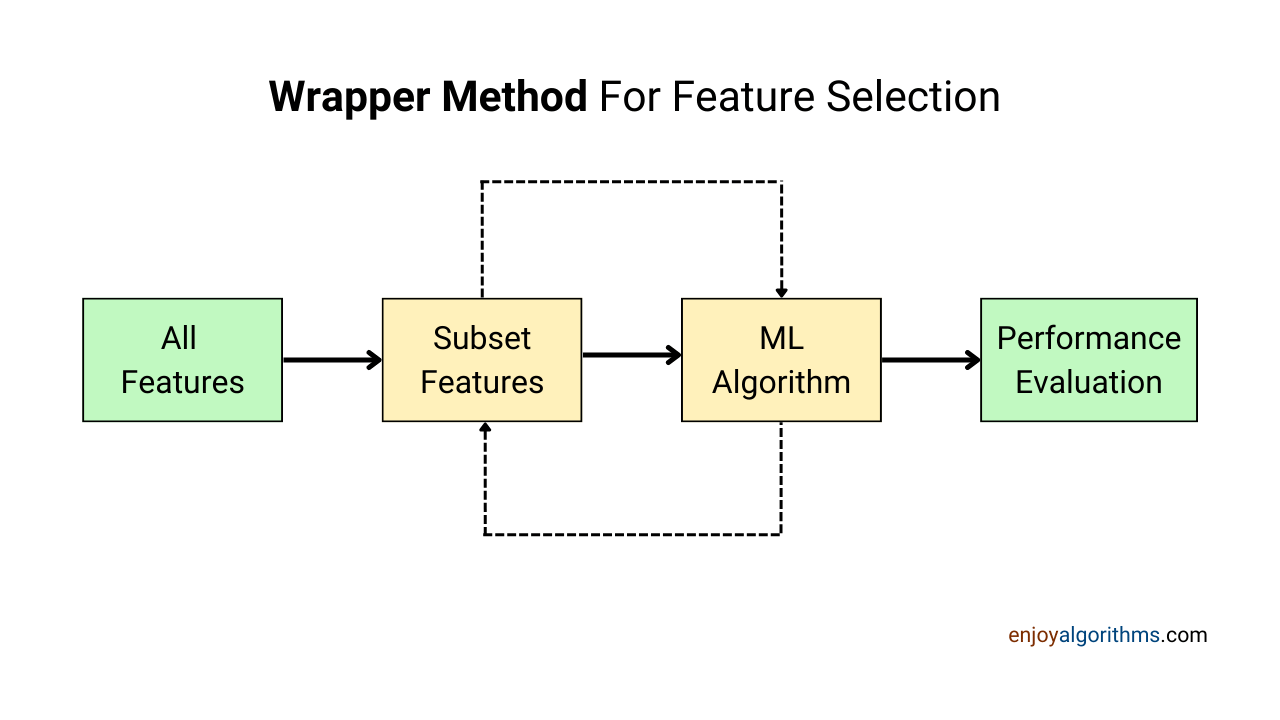Let's look at some wrapper feature selection techniques:

#### Forward Feature Selection

This method works iteratively by selecting the best variable among all the features and clubs another variable with the previously selected variable. This process persists until a specific criterion is fulfilled. Let's implement Forward Feature Selection on the Boston house price dataset:

``````import numpy as np
import pandas as pd

from mlxtend.feature_selection import SequentialFeatureSelector

from sklearn.linear_model import LinearRegression

features = boston_house_price.iloc[:,:13]

target = boston_house_price.iloc[:,-1]

SFS = SequentialFeatureSelector(LinearRegression(),  #Regressor
k_features=12,       #When to stop
forward=True,        #Ensures FFS
scoring = 'r2')      #Scoring metric

SFS.fit(features, target)
SFS_results = pd.DataFrame(SFS.subsets_).transpose()

SFS_results``````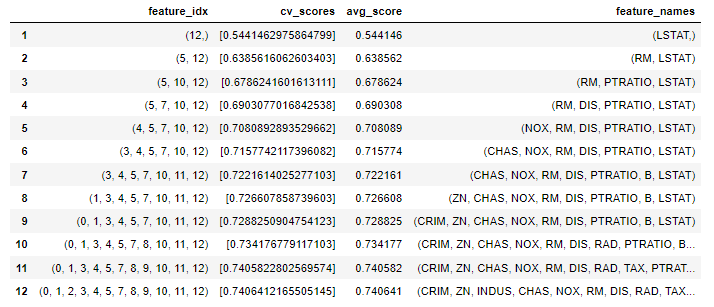In the above illustration, we are using the Boston House Price dataset. It's a regression problem; hence, we are utilizing the linear regression model to fit the data. R-squared error is a performance metric here. The model's performance rapidly increased till the top seven features, and then it saturated around 0.74 avg_score. These results indicate that the seven features are sufficient for building the model, and the rest of the features can be dumped to keep the model explainable and faster.

#### Backward Feature Elimination

Backward Feature Elimination is just the opposite of the above method. We start with all the features and fit the model. Then, we eliminate the feature from the model to which we receive the best performance. This process is repeated till we achieve a specific criterion. In the case below, the stopping criteria would be to halt once we were left with four parameters only. We can adjust the stopping criteria accordingly.

``````from mlxtend.feature_selection import SequentialFeatureSelector

from sklearn.linear_model import LinearRegression

import pandas as pd
import numpy as np

features = boston_house_price.iloc[:,:13]
target = boston_house_price.iloc[:,-1]

SFS = SequentialFeatureSelector(LinearRegression(),  #Regressor
k_features=4,        #When to stop
forward=False,       #Ensures BFE
scoring = 'r2')      #Scoring metric

SFS.fit(features, target)
SFS_results = pd.DataFrame(SFS.subsets_).transpose()

SFS_results``````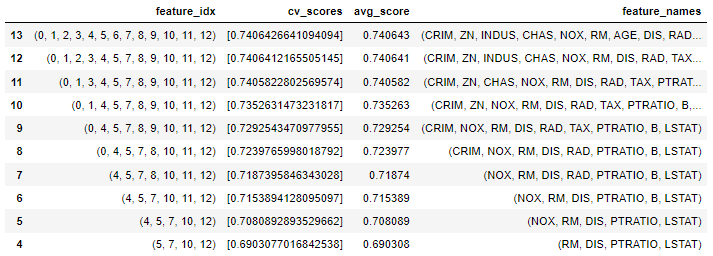#### Exhaustive Feature Selection (EFS)

This method searches for all possible combinations of features and evaluates the model over each subset of features. The output of EFS would be the combination of features securing the best score. It is a brute-force approach with high computational time. Let's implement it over the Boston house price prediction dataset.

``````from mlxtend.feature_selection import ExhaustiveFeatureSelector as EFS
from sklearn.linear_model import LinearRegression

lr = LinearRegression()

features = boston_house_price.iloc[:,:13]
target = boston_house_price.iloc[:,-1]

# Create an EFS object
efs = EFS(LinearRegression(),     # Regressor
min_features=6,       # Min features to consider
max_features=13,      # Max features to consider
scoring='r2')         # R-Squared as evaluation criteria

# Train EFS with our dataset
efs = efs.fit(features, target)

# Print the results
print('Best subset (indices):', efs.best_idx_)
print('Best subset (corresponding names):', efs.best_feature_names_)

# Best subset (indices): (0, 1, 3, 4, 6, 7, 8, 9, 10, 11, 12)
# Best subset (corresponding names): ('CRIM', 'ZN', 'CHAS', 'NOX', 'AGE', 'DIS',
#                   'RAD', 'TAX', 'PTRATIO', 'B', 'LSTAT')``````

Now that we have understood the wrapper method working let's look at the other way,

### Embedded Method

The embedded method surpasses the filter and wrapper methods due to its fair computational cost and reliable performance. Embedded methods are algorithm-based, where an algorithm helps extract the relevant features. The algorithm keeps track of relevant features using certain criteria and collects the most contributing features during the training phase.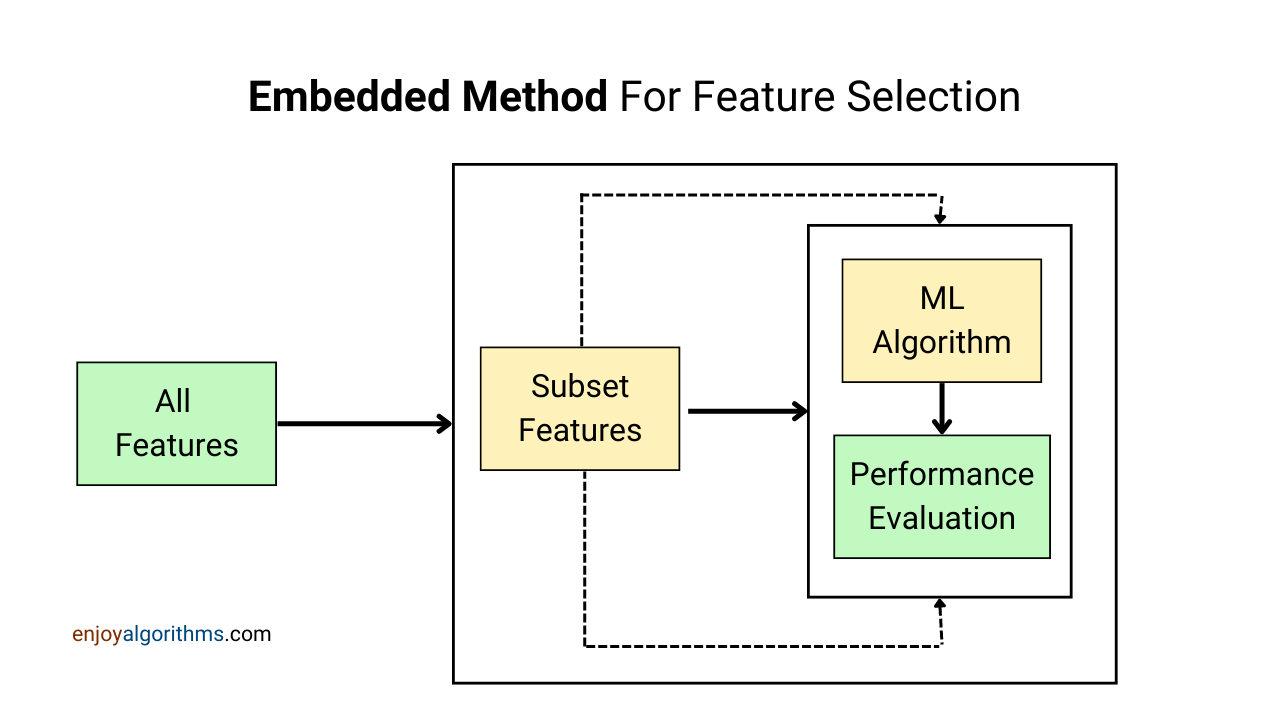The computational cost for the embedded method is lower than the wrapper methods, and performance is remarkably better than the other two methods. Let's look at some embedded feature selection techniques:

#### LASSO Regularization L1

LASSO Regularization is commonly used as a feature selection criterion. It penalizes irrelevant parameters by shrinking their weights or coefficients to zero. Hence, those features are removed from the model, and it not only removes the extraneous features and prevents the model from overfitting. One can learn the complete working of regularization in our blog of Regularization: A fix to overfitting.

Let's implement LASSO Regularization:

``````from sklearn.linear_model import LogisticRegression
from sklearn.feature_selection import SelectFromModel
from sklearn.preprocessing import StandardScaler

target = diabetes["Outcome"]
features = diabetes.drop("Outcome", axis=1)

scaler = StandardScaler()
scaler.fit(features)
scaled_features = scaler.transform(features)

logistic = SelectFromModel(LogisticRegression(C=1, penalty='l1', solver='liblinear'))
logistic.fit(scaled_features, target)

selected_features = features.columns[(logistic.get_support())]

print('Total number of features: {}'.format((features.shape)))
print('Features selected: {}'.format(len(selected_features)))
print('Number of discarded features: {}'.format(np.sum(logistic.estimator_.coef_ == 0)))

# Total number of features: 8
# Features selected: 7
# Number of discareded features: 1``````
``````features.columns[(logistic.estimator_.coef_ == 0).ravel()]

# Index(['SkinThickness'], dtype='object')``````

#### Random Forest Feature Importance

Random Forest falls under ensemble learning algorithms that utilize several weak learners' aggregation (decision trees) for prediction. This tree-based approach naturally ranks the features of a dataset by measuring how well the purity is improving. In the decision trees, the impurity drops rapidly at the starting node of the tree, and this rate decreases as we go down. Naturally, the initial node of the tree holds more critical Information. Hence, such features are relevant from the feature selection perspective, while those contributing to the lower portion of the tree are less relevant. This mechanism allows us to create a hierarchy of features sorted by importance. Let's implement Random Forest Feature Importance:

``````from sklearn.ensemble import RandomForestClassifier

features = df.drop('Outcome',axis=1)
target = df['Outcome']

classifier = RandomForestClassifier(random_state=90, oob_score=True)
classifier.fit(features, target)

feature_importance = classifier.feature_importances_

feature_importance = 100.0 * (feature_importance / feature_importance.max())

sorted_idx = np.argsort(feature_importance)
sorted_idx = sorted_idx[len(feature_importance) - 50:]

pos = np.arange(sorted_idx.shape) + .5

plt.figure(figsize=(10,12))
plt.barh(pos, feature_importance[sorted_idx], align='center')
plt.xticks(size =14)
plt.yticks(pos, features.columns[sorted_idx], size =14)
plt.xlabel('Relative Importance', fontsize = 15)
plt.title('Variable Importance', fontsize = 15)
plt.show()``````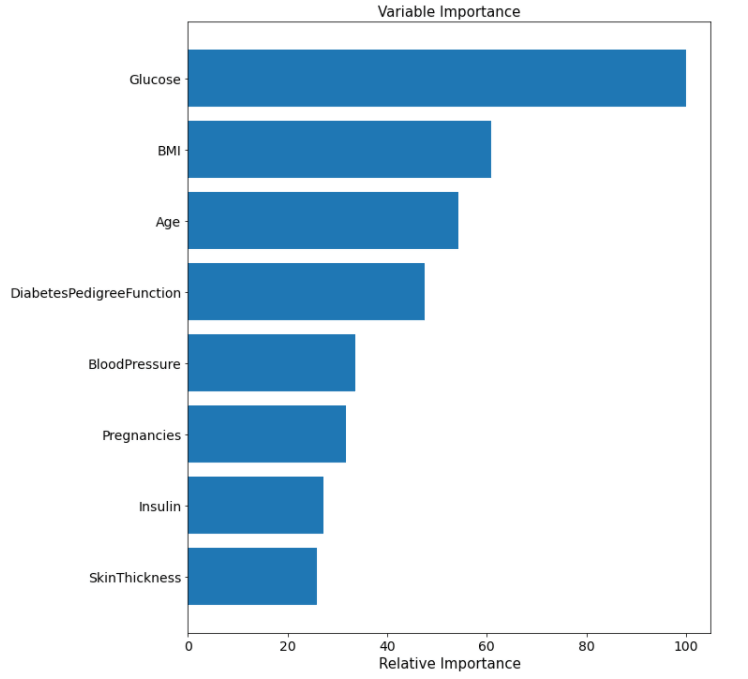### Filter Method

Filter methods are a collection of statistical techniques commonly used for measuring the importance of features in a dataset. These methods are fast and computationally inexpensive than the wrapper method. While dealing with large datasets, it is more reasonable to use filter methods. Let's look at some filter feature selection methods:

#### Correlation Coefficients

Correlation helps measure the linear relationship between two or more features and is primarily valid when features are numeric. Its application extends as a feature selection method since the correlation matrix, a.k.a the heatmap, helps visualize the relationship between the features and the target variable. It can also reveal Information on collinear features, which are redundant in the analysis since they don't contribute to any new information; thus, removing such features is recommended. Secondly, we can decide on a threshold value of correlation. If the absolute correlation between the feature and target variable is lower than that threshold, we can discard that feature from the analysis. Let's implement a correlation heatmap:

``````import seaborn as sns
import matplotlib.pyplot as plt

correlation = boston_house_price.corr()
plt.figure(figsize= (15,12))
sns.heatmap(correlation, annot=True)``````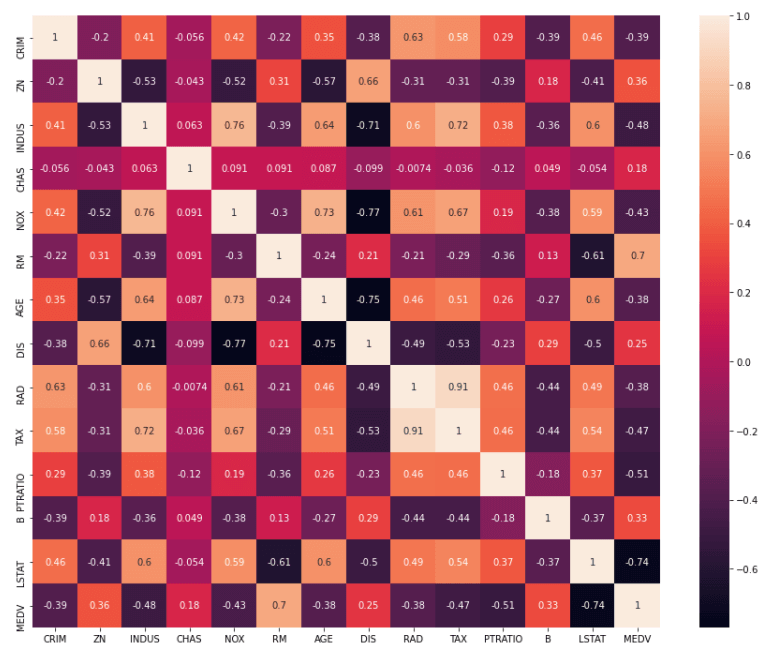TAX and RAD parameters share a high correlation. Keeping anyone of TAX and RAD would suffice. Now, which parameter should we remove out of TAX and RAD? For this, we will check the absolute correlation with the target variable. The target variable is MEDV and TAX has a high absolute correlation with MEDV. Hence, we will discard RAD from the analysis.

We also need to decide on a threshold of absolute correlation. Below this threshold, we will toss the feature from the analysis. Let's keep it 0.4 as a feature selection criterion. We are left with 'INDUS,' 'NOX,' 'RM,' 'TAX,' 'PTRATIO,' and 'LSTAT.' as the final features. Selecting an optimal threshold is an empirical process and requires hit & trial to arrive at an optimum threshold value.

#### Mutual Information

Mutual Information is a feature selection technique commonly used when the independent features are numeric. It measures the dependency of an independent variable over the target variable. The Mutual Information is zero when two variables are independent, and a higher value suggests a higher dependence. It relies on entropy estimation, which further uses the K-nearest-neighbors distances. Mutual Information applies to both regression and classification problems. Let's implement this over the wine-quality dataset.

``````import pandas as pd
from sklearn.feature_selection import mutual_info_classif

target = wine_quality["quality"]

features = wine_quality.drop("quality", axis=1)

mutual_information = mutual_info_classif(features, target)
mutual_information_series = pd.Series(mutual_information)

mutual_information_series.index = features.columns

mutual_information_series.sort_values(ascending=False)
mutual_information_series.sort_values(ascending=False).plot.bar()``````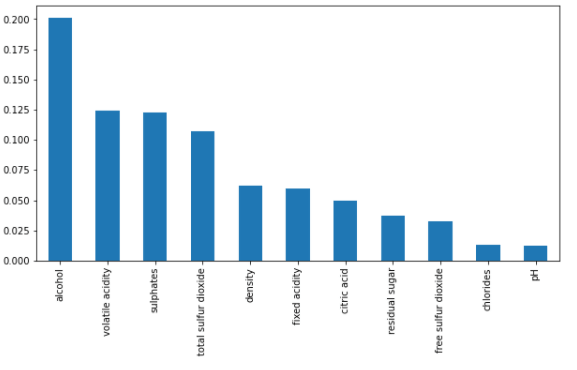The initial nine parameters in descending order have a significant relationship with the dependent parameter. The rest of the parameters can be scrapped from the analysis.

#### Variance Threshold

This approach assumes that the features with low variance do not contribute much Information to the analysis. Variables with zero variance are the first to be removed; such variables contain a single input throughout the dataset and provide no valuable information. Features with high variance are often helpful as per this method, but this is only true for some instances. A variance threshold is required as a feature selection criterion. Any features having variance lower than the decided threshold will be tossed.

``````from sklearn.feature_selection import VarianceThreshold

target = wine_quality["quality"]
features = wine_quality.drop("quality", axis=1)

selector = VarianceThreshold(threshold=0.03)
selector.fit(features)

best_features = features.columns[selector.get_support()]
print(best_features)

## Index(['fixed acidity', 'volatile acidity', 'citric acid', 'residual
## sugar', 'free sulfer dioxide', 'total sulfer dioxide', 'alcohol'], dtype='object')``````

#### Chi-Square Test

The chi-Squared test is used when all the parameters are categorical in the dataset. It requires the computation of the chi-square value between the feature and the target. Features are selected based on their chi-square scores. Before applying the chi-square test, certain conditions have to be met. Following are those conditions:

• Features should be categorical
• Observations should be independent
• The sample should be large (features having a frequency greater than 5)

Let's implement the Chi-Square Test:

``````from sklearn.feature_selection import SelectKBest, chi2
from sklearn.preprocessing import LabelEncoder

le = LabelEncoder()
cat_columns = tennis_data.columns

tennis_data[cat_columns] = tennis_data[cat_columns].apply(lambda x: le.fit_transform(x))

target = tennis_data["play"]
features = tennis_data.drop(["play"], axis=1)

chi2_features = SelectKBest(chi2, k = 4)
Best_k_features = chi2_features.fit_transform(features, target)

print("Total Number of Features" ,features.shape)
print("Reduced to {} features".format(Best_k_features.shape))

## Total Number of Features 5
## Reduced to 4 features``````

### Possible Interview Questions

Feature selection is a common topic that interviewers like to ask about because it is often a part of the projects mentioned on resumes. Some questions that may be asked include:

• How many features were there in the raw data you received?
• What was the final number of features used to train the machine learning model?
• How did you decide to remove certain features from the final set of features?
• If the raw data had 1000 features and a large number of samples, which method would you prefer?
• Why is a wrapper function considered a greedy approach?

### Conclusion

In this article, we examined three main methods for feature selection: Wrapper, Embedded, and Filter. Each of these methods has its own advantages and disadvantages, and there is no one perfect method for feature selection. The best features identified by each method can vary, and selecting the most effective features is a trial-and-error process that requires data experimentation and an understanding of the domain.

We implemented all of these strategies in Python and gained an understanding of the underlying principles behind each technique. Filter methods are efficient and work well with large datasets, while wrapper methods are reliable but slower and better suited for small datasets. The embedded method takes an intermediate amount of time to run compared to the wrapper and filter methods, but it produces reliable results.

##### Enjoy Learning, Enjoy Algorithms!

☆ 16-Week Live DSA Course
☆ 10-Week Live DSA Course